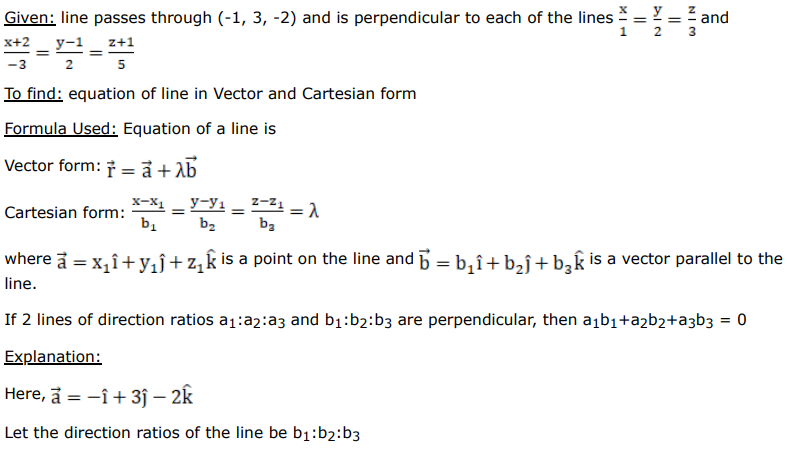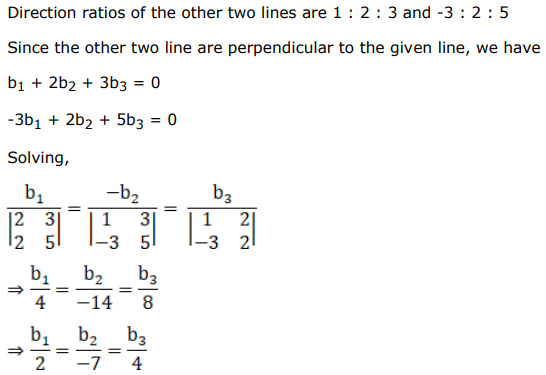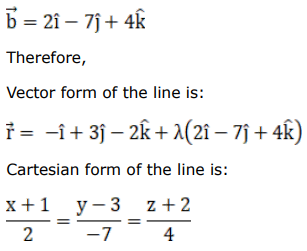# Find the equations of the line passing through the point

Question:

Find the equations of the line passing through the point $(-1,3,-2)$ and perpendicular to each of the lines $\frac{x}{1}=\frac{y}{2}=\frac{z}{3}$ and $\frac{x+2}{-3}=\frac{y-1}{2}=\frac{z+1}{5}$.

Solution: